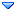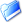GSI Forum
GSI Helmholtzzentrum für Schwerionenforschung

Home » PANDA » PandaRoot » Analysis » Tutorial feb2012
Tutorial feb2012 Thu, 10 May 2012 11:01Dmitry Khaneft Messages: 75Registered: January 2009 continuous participant From: *kph.uni-mainz.de
Dear all,

I try to follow tutorial available at feb12.

I slightly modified it for my needsand try to plot some simple distributions but I can't see an effect of applying different PID criteria.

For example: All, VeryLoose, Loose, Tight, VeryTight give same results.

Here is a part of my code.

```// *** TCandLists for the analysis
TCandList eplus, eminus;

...

while (theAnalysis->GetEvent() && i++<nevts){
if ((i%1000)==0) cout << "Number of events analysed: " << i << endl;

// *** Select with no PID info ('All'); type and mass are set
theAnalysis->FillList(eplus, "ElectronTightPlus");
//theAnalysis->FillList(eplus, "ElectronTightPlus","PidAlgoEmcBayes");
theAnalysis->FillList(eminus, "ElectronTightPlus");
//theAnalysis->FillList(eminus, "ElectronTightMinus","PidAlgoEmcBayes");

// ELECTRONS ***
for (Int_t j=0;j<eminus.GetLength();++j){
// Fill momentum of reconstructed particles
hP->Fill(eminus[j].GetMomentum().Mag());
// Fill energy of reconstructed particles
hE->Fill(eminus[j].Energy());
// Fill momentum vs E/p
hEP->Fill(eminus[j].GetMomentum().Mag(), eminus[j].Energy()/eminus[j].GetMomentum().Mag());
// *** CM frame *** //
// boost from LAB to CM
lv_charged_cm.SetVect(eminus[j].GetMomentum());
lv_charged_cm.SetE(TMath::Sqrt(cElectronMass*cElectronMass + eminus[j].GetMomentum().Mag()*eminus[j].GetMomentum().Mag()));
lv_charged_cm.Boost(-(lv_bar_beam + lv_p_target).BoostVector());

hCosTheta_N_CM->Fill(lv_charged_cm.CosTheta());
}
for (Int_t j=0;j<eplus.GetLength();++j){
// Fill momentum of reconstructed particles
hP->Fill(eplus[j].GetMomentum().Mag());
// Fill energy of reconstructed particles
hE->Fill(eplus[j].Energy());
// Fill momentum vs E/p
hEP->Fill(eplus[j].GetMomentum().Mag(), eplus[j].Energy()/eplus[j].GetMomentum().Mag());
// *** CM frame *** //
// boost from LAB to CM
lv_charged_cm.SetVect(eplus[j].GetMomentum());
lv_charged_cm.SetE(TMath::Sqrt(cElectronMass*cElectronMass + eplus[j].GetMomentum().Mag()*eplus[j].GetMomentum().Mag()));
lv_charged_cm.Boost(-(lv_bar_beam + lv_p_target).BoostVector());

hCosTheta_P_CM->Fill(lv_charged_cm.CosTheta());
}
}
```

In addition histograms filled with energy and momentum are the same. Using "PidAlgoEmcBayes" also gives no effect.

Is it suppose to be like this or I do something wrong?

P.S. I used trunk 15407 instead of 14709 because later couldn't be compiled.

Cheers,
DmitryTutorial feb2012 By: Dmitry Khaneft on Thu, 10 May 2012 11:01Re: Tutorial feb2012 By: StefanoSpataro on Thu, 10 May 2012 11:42Re: Tutorial feb2012 By: Dmitry Khaneft on Thu, 10 May 2012 12:08Re: Tutorial feb2012 By: StefanoSpataro on Thu, 10 May 2012 12:14Re: Tutorial feb2012 By: Dmitry Khaneft on Thu, 10 May 2012 12:19Re: Tutorial feb2012 By: StefanoSpataro on Thu, 10 May 2012 12:29Re: Tutorial feb2012 By: Ralf Kliemt on Thu, 10 May 2012 12:53Re: Tutorial feb2012 By: StefanoSpataro on Thu, 10 May 2012 13:35Re: Tutorial feb2012 By: Klaus Götzen on Thu, 10 May 2012 15:39
 Previous Topic: negative chi2 in PndKinVtxFitter ! Next Topic: editing pidana.par
Goto Forum:

Current Time: Tue Nov 30 04:15:37 CET 2021

Total time taken to generate the page: 0.02413 seconds